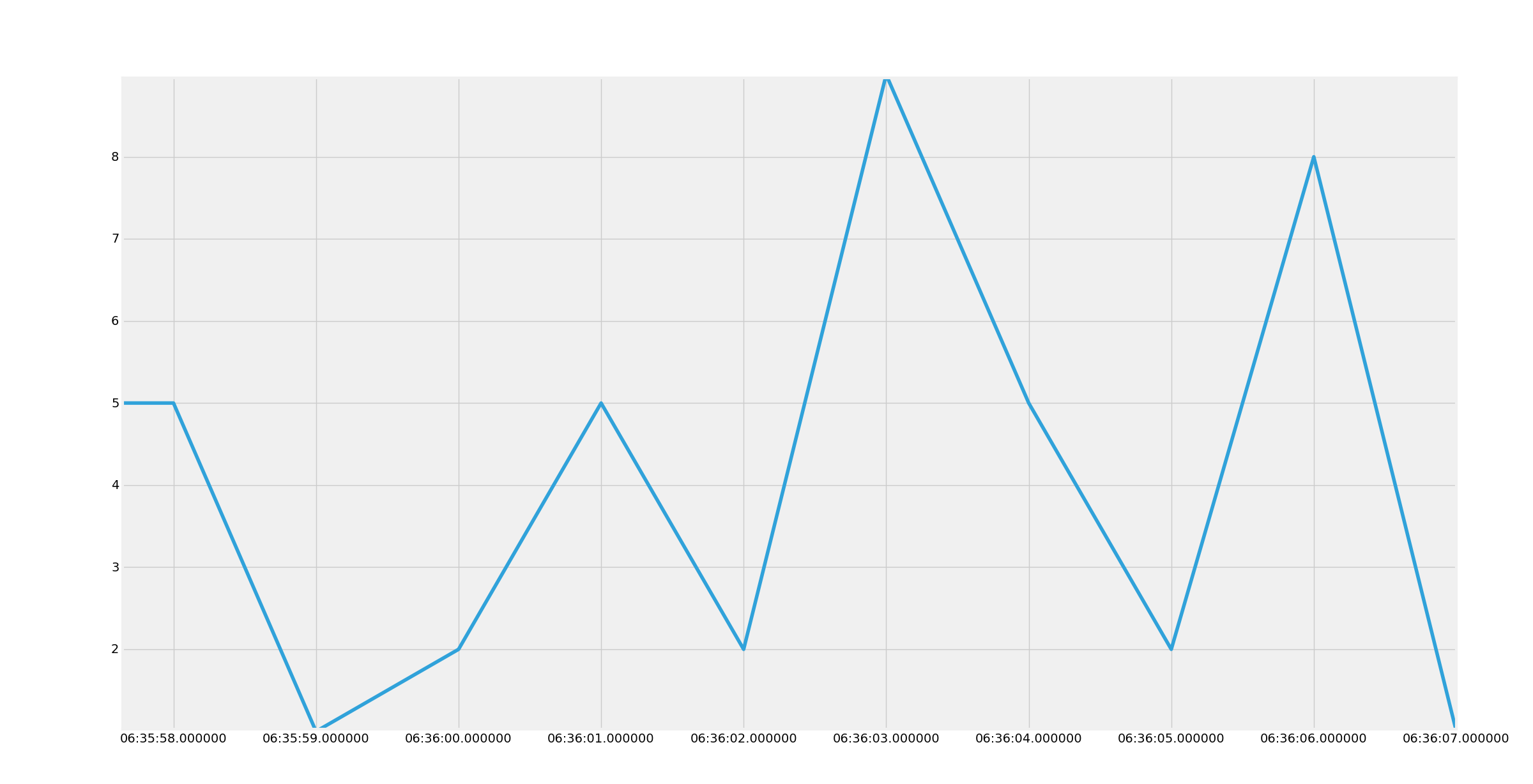## Graphing example from SQLite

In this tutorial, we're going to show how you can use a select query, and iterate through it, to get data that you can make use of. In this example, we're going to generate a Matplotlib graph. Check out that tutorial regarding how to get Matplotlib if you do not already have it.

Code up to this point:

```import sqlite3
import time
import datetime
import random

conn = sqlite3.connect('tutorial.db')
c = conn.cursor()

def create_table():
c.execute("CREATE TABLE IF NOT EXISTS stuffToPlot(unix REAL, datestamp TEXT, keyword TEXT, value REAL)")

def data_entry():
c.execute("INSERT INTO stuffToPlot VALUES(1452549219,'2016-01-11 13:53:39','Python',6)")

conn.commit()
c.close()
conn.close()

def dynamic_data_entry():

unix = int(time.time())
date = str(datetime.datetime.fromtimestamp(unix).strftime('%Y-%m-%d %H:%M:%S'))
keyword = 'Python'
value = random.randrange(0,10)

c.execute("INSERT INTO stuffToPlot (unix, datestamp, keyword, value) VALUES (?, ?, ?, ?)",
(unix, date, keyword, value))

conn.commit()
time.sleep(1)

c.execute('SELECT * FROM stuffToPlot')
data = c.fetchall()
print(data)
for row in data:
print(row)

c.execute('SELECT * FROM stuffToPlot WHERE value = 3')
data = c.fetchall()
print(data)
for row in data:
print(row)

c.execute('SELECT * FROM stuffToPlot WHERE unix > 1452554972')
data = c.fetchall()
print(data)
for row in data:
print(row)

c.execute('SELECT value, datestamp FROM stuffToPlot WHERE unix > 1452554972')
data = c.fetchall()
print(data)
for row in data:
print(row)

c.close
conn.close()```

Now we will make another function, called `graph_data`:

```def graph_data():
c.execute('SELECT datestamp, value FROM stuffToPlot')
data = c.fetchall()

dates = []
values = []

for row in data:
dates.append(parser.parse(row))
values.append(row)

plt.plot_date(dates,values,'-')
plt.show()```

In this example, we're grabbing the datestamp, and the value from the table. From there, we're iterating through the return to popluate a `dates` and `values` list. After that, we use Matplotlib to plot the data. This means we probably need to import Matplotlib! Add the following to the top:

```import matplotlib.pyplot as plt
import matplotlib.dates as mdates
from dateutil import parser
from matplotlib import style
style.use('fivethirtyeight')```

Run that, and you should get a graph like:Your graph will be a different line, since our numbers were randomly generated.

Next, we're going to cover how to modify existing data and how to delete from the database table.